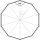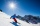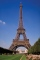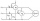# Tangent - high school - math problems

Tangent is a trigonometric function. In a rectangular triangle, it is the ratio of the opposite and adjacent side to a given internal angle. Algebraically is defined as the ratio of the sine and cosine of a given angle. It is periodic with a period of π = 180 °.

#### Number of problems found: 98

• WhichWhich of the following numbers most accurately area of a regular decagon with side s = 2 cm? (A) 9.51 cm2 (B) 20 cm2 (C) 30.78 cm2 (D) 31.84 cm2 (E) 32.90 cm2Calculate the content of a regular 15-sides polygon inscribed in a circle with radius r = 4. Express the result to two decimal places.
• Powerplant chimneyFrom the window of the building at a height of 7.5 m, the top of the factory chimney can be seen at an altitude angle of 76° 30 ′. The base of the chimney can be seen from the same place at a depth angle of 5° 50 ′. How tall is the chimney?
• The rescue helicopterThe rescue helicopter is above the landing site at a height of 180m. The site of the rescue operation can be seen from here at a depth angle of 52° 40 '. How far will the helicopter land from the rescue site?
• Traffic signThere is a traffic sign for climbing on the road with an angle of 7%. Calculate at what angle the road rises (falls).
• FighterA military fighter flies at an altitude of 10 km. From the ground position, it was aimed at an altitude angle of 23° and 12 seconds later at an altitude angle of 27°. Calculate the speed of the fighter in km/h.
• Black diamond runTaleah is skiing down a black diamond run. She begins skiing at the top of a ski trail whose elevation is about 8625 feet. The ski run ends toward the base of the mountain at 3800 feet. The horizontal distance between these two points is about 4775 feet.
• The towerThe observer sees the base of the tower 96 meters high at a depth of 30 degrees and 10 minutes and the top of the tower at a depth of 20 degrees and 50 minutes. How high is the observer above the horizontal plane on which the tower stands?
• An observerAn observer standing west of the tower sees its top at an altitude angle of 45 degrees. After moving 50 meters to the south, he sees its top at an altitude angle of 30 degrees. How tall is the tower?
• Tetrahedral pyramid 8Let’s all side edges of the tetrahedral pyramid ABCDV be equally long and its base let’s be a rectangle. Determine its volume if you know the deviations A=40° B=70° of the planes of adjacent sidewalls and the plane of the base and the height h=16 of the pCalculate the volume (V) and the surface (S) of a regular quadrilateral prism whose height is 28.6 cm and the deviation of the body diagonal from the base plane is 50°.
• Power line poleFrom point A, the power line pole is seen at an angle of 18 degrees. From point B to which we get when going from point A 30m away from the column at an angle of 10 degrees. Find the height of the power pole.
• Cone sideCalculate the volume and area of the cone whose height is 10 cm and the axial section of the cone has an angle of 30 degrees between height and the cone side.
• The Eiffel TowerThe top of the Eiffel Tower is seen from a distance of 600 meters at an angle of 30 degrees. Find the tower height.
• Tetrahedral pyramidDetermine the surface of a regular tetrahedral pyramid when its volume is V = 120 and the angle of the sidewall with the base plane is α = 42° 30´.
• Octagonal pyramidFind the volume of a regular octagonal pyramid with height v = 100 and the angle of the side edge with the plane of the base is α = 60°.
• Isosceles triangle 10In an isosceles triangle, the equal sides are 2/3 of the length of the base. Determine the measure of the base angles.
• Depth angleFrom a cliff of 150 meters high, we can see the ship at a depth angle of 9° at sea. How far is the ship from the cliff?Two wattmeters are connected to measuring power in a 3 phase balanced load. Determine the total power and power factor if the two wattmeters read 1000 watts each (1) both positive and (2) second reading is negativeThere is a regular quadrangular pyramid ABCDV; | AB | = 4 cm; height v = 6 cm. Determine the angles of lines AD and BV.